# 1. 下载与安装

（1） 下载

https://www.boost.org/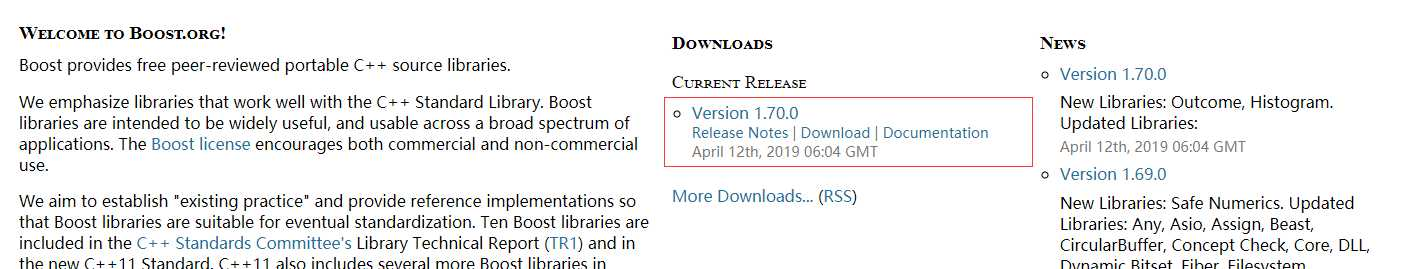（2）配置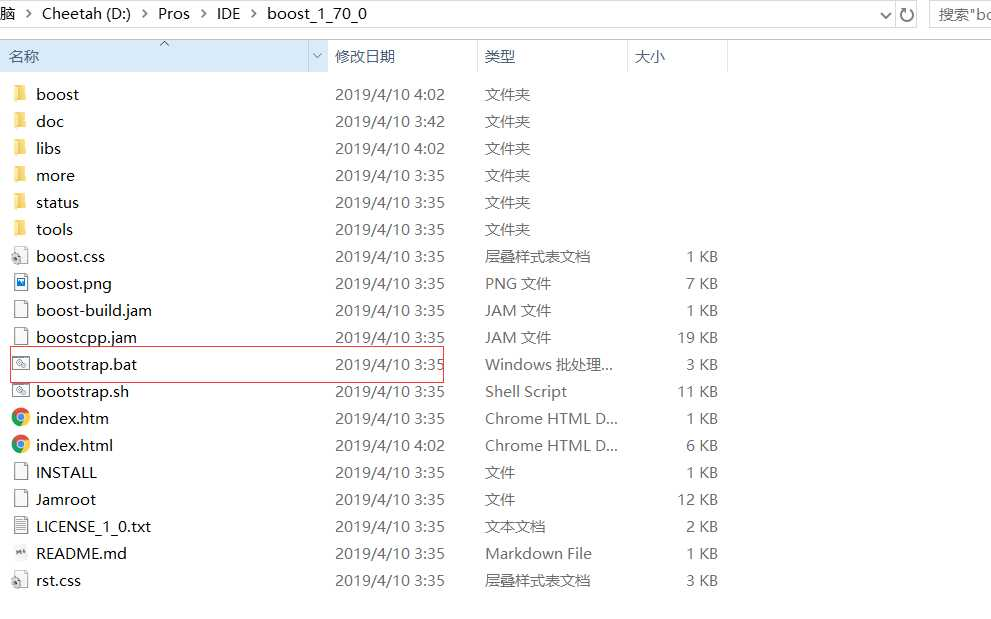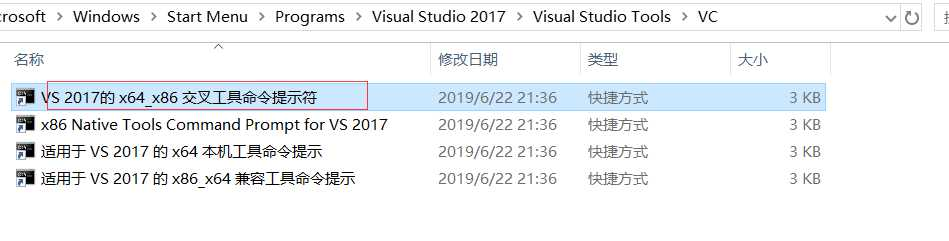（3）执行后，将会在boost当前目录下增加了几个文件，找到其中的bjam.exe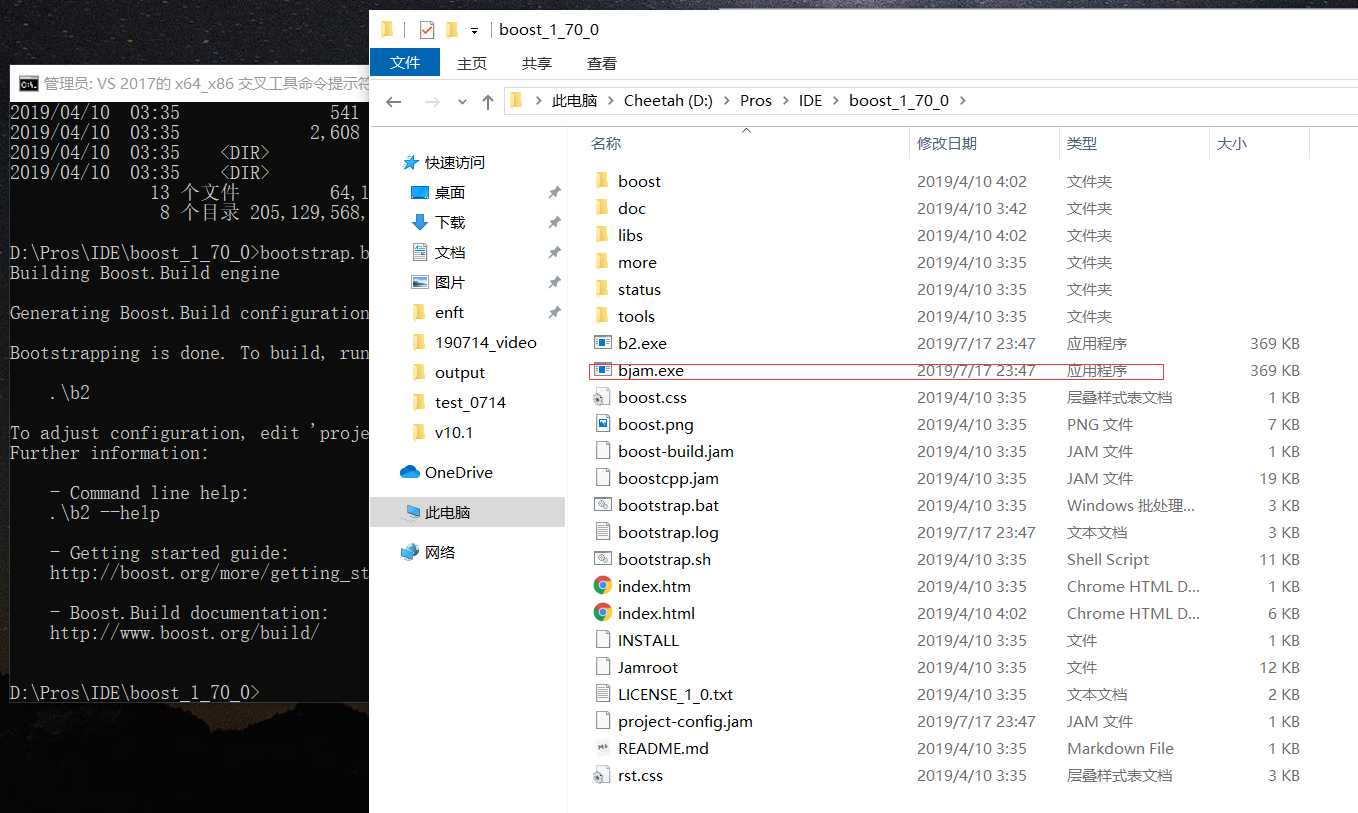（4）生成 包含目录和库目录

Note:

MSVC 版本号对应：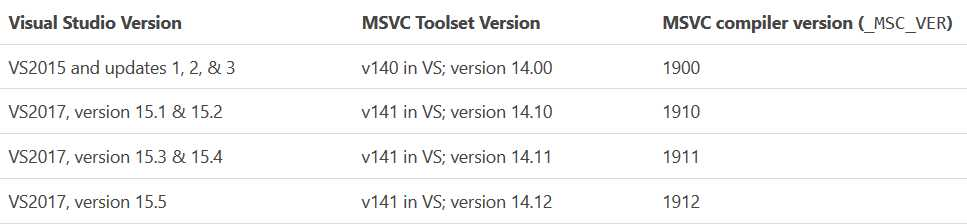1. MSVC++ 14.1 _MSC_VER == ??00 (Visual Studio 2017)
2. MSVC++ 14.0 _MSC_VER == 1900 (Visual Studio 2015)
3. MSVC++ 12.0 _MSC_VER == 1800 (Visual Studio 2013)
4. MSVC++ 11.0 _MSC_VER == 1700 (Visual Studio 2012)
5. MSVC++ 10.0 _MSC_VER == 1600 (Visual Studio 2010)
6. MSVC++ 9.0  _MSC_VER == 1500 (Visual Studio 2008)
7. MSVC++ 8.0  _MSC_VER == 1400 (Visual Studio 2005)
8. MSVC++ 7.1  _MSC_VER == 1310 (Visual Studio 2003)
9. MSVC++ 7.0  _MSC_VER == 1300
10. MSVC++ 6.0  _MSC_VER == 1200
11. MSVC++ 5.0  _MSC_VER == 1100

vs2017 可以支持boost c++ library 针对msvc-14.1的编译选项。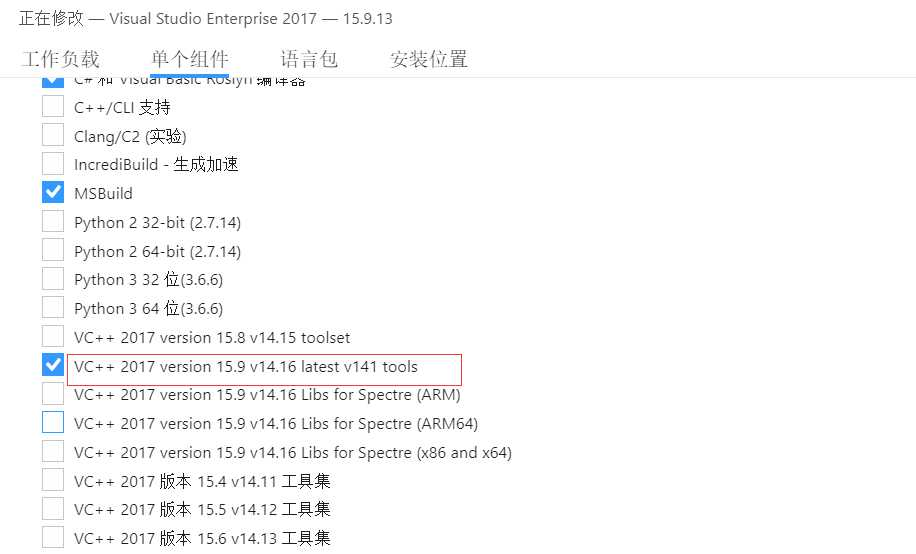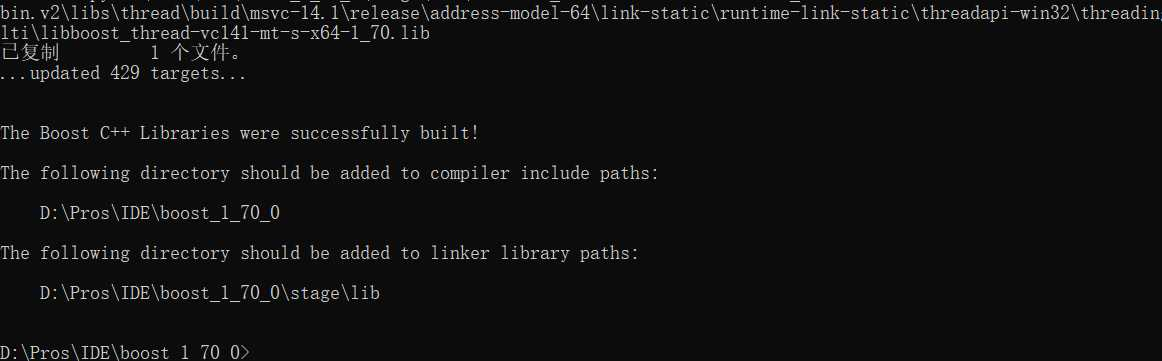这时boost库已经安装、编译成功。

# 2. 测试

（1）新建vs2017 c++项目，并添加boost依赖库

（2）代码

```#include <boost/lexical_cast.hpp>
#include <iostream>

using namespace std;
using namespace boost;

int main()
{
//system("chcp 65001");

double a = lexical_cast<double>("3.1415926");
string str = lexical_cast<string>("3.1415926");
cout << "This is a number: " << a << endl;
cout << "This is a string: " << str << endl;
int b = 0;
try {
b = lexical_cast<int>("neo");
}
cout << e.what() << endl;
}
return 0;
}
```

# 3、boost库的说明

c++ boost库官网

Boost库是一个可移植、提供源代码的C++库，作为标准库的后备，是C++标准化进程的开发引擎之一，是为C++语言标准库提供扩展的一些C++程序库的总称

Boost库由C++标准委员会库工作组成员发起，其中有些内容有望成为下一代C++标准库内容。在C++社区中影响甚大，是不折不扣的“准”标准库。Boost由于其对跨平台的强调，对标准C++的强调，与编写平台无关。大部分boost库功能的使用只需包括相应头文件即可，少数（如正则表达式库，文件系统库等）需要链接库。但Boost中也有很多是实验性质的东西，在实际的开发中使用需要谨慎。

Boost社区建立的初衷之一就是为C++的标准化工作提供可供参考的实现，Boost社区的发起人Dawes本人就是C++标准委员会的成员之一。在Boost库的开发中，Boost社区也在这个方向上取得了丰硕的成果。在送审的C++标准库TR1中，有十个Boost库成为标准库的候选方案。在更新的TR2中，有更多的Boost库被加入到其中。从某种意义上来讲，Boost库成为具有实践意义的准标准库。

## 3.1 主要分类

a) Conversion
b) Format
c) IOStream
d) Lexical Cast
e) Regex
f) Spirit
g) String Algo
h) Tokenizer
i) Wave
j) Xpressive

a) Array
b) Bimap
c) Circular Buffer
d) Disjoint Sets
e) Dynamic Bitset
f) GIL
g) Graph
h) ICL
i) Intrusive
j) Multi-Array
k) Multi-Index
l) Pointer Container
m) Property Map
n) Property Tree
o) Unordered
p) Variant

a) GIL
b) Graph
c) Iterators
d) Operators
e) Tokenizer

a) Foreach
b) GIL
c) Graph
d) Min-Max
e) Range
f) String Algo
g) Utility

a) Bind
b) Function
c) Functional
d) Functional/Factory
e) Functional/Forward
f) Functional/Hash
g) Lambda
h) Member Function
i) Ref
j) Result Of
k) Signals
l) Signals2
m) Utility

a) Call Traits
b) Concept Check
c) Enable If
d) Function Types
e) GIL
f) In Place Factory, Typed In Place Factory
g) Operators
h) Property Map
i) Static Assert
j) Type Traits

a) Function Types
b) Fusion
c) MPL
d) Proto
e) Static Assert
f) Type Traits

a) Preprocessors

a) Asio
b) Interprocess
c) MPI

a) Accumulators
b) Integer
c) Interval
d) Math
e) Math Common Factor
f) Math Octonion
g) Math Quaternion
h) Math/Special Functions
i) Math/Statistical Distributions
j) Multi-Array
k) Numeric Conversion
l) Operators
m) Random
n) Rational
o) uBLAS

a) Concept Check
b) Static Assert
c) Test

a) Any
b) Bitmap
c) Compressed Pair
d) Fusion
e) ICL
f) Multi-Index
g) Pointer Container
h) Property Tree
i) Tuple
j) Uuid
k) Variant

a) GIL

a) Asio
b) Assign
c) Format
d) IO State Savers
e) IOStreams
f) Program Options
g) Serialization

a) Python

a) Pool
b) Smart Ptr
c) Utility

a) Spirit

a) Function
b) Parameter

a) Compressed Pair
b) Conversion
c) CRC
d) Date Time
e) Exception
f) Filesystem
g) Flyweight
h) Lexical Cast
i) Meta State Machine
j) Numeric Conversion
k) Optional
l) Polygon
m) Program Options
n) Scope Exit
o) Statechart
p) Swap
q) System
r) Timer
s) Tribool
t) Typeof
u) Units
v) Utility
w) Value Initialized

a) Compatibility
b) Config

## 3.2 常用库

Regex

Spirit
LL parser framework，用C++代码直接表达EBNF
Graph

Lambda

concept check

Mpl

Python

Pool

smart_ptr
5个智能指针，学习智能指针必读，一份不错的参考是来自CUJ的文章：

Boost程序库完全开发指南：深入C++“准”标准库
C++11/14高级编程：Boost程序库探秘（第3版）
Boost程序库探秘：深度解析C++准标准库

VS2017 配置 boost_1_70

(0)
(0)